2022: SklogWiki celebrates 15 years on-line

# Heat capacity

The heat capacity is defined as the differential of heat with respect to the temperature$T$,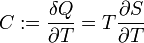$C := \frac{\delta Q}{\partial T} = T \frac{\partial S}{\partial T}$

where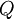$Q$ is heat and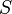$S$ is the entropy.

## At constant volume

From the first law of thermodynamics one has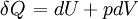$\left.\delta Q\right. = dU + pdV$

thus at constant volume, denoted by the subscript$V$, then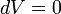$dV=0$,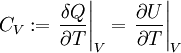$C_V := \left.\frac{\delta Q}{\partial T} \right\vert_V = \left. \frac{\partial U}{\partial T} \right\vert_V$

## At constant pressure

At constant pressure (denoted by the subscript$p$),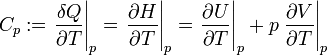$C_p := \left.\frac{\delta Q}{\partial T} \right\vert_p =\left.\frac{\partial H}{\partial T} \right\vert_p= \left. \frac{\partial U}{\partial T} \right\vert_p + p \left.\frac{\partial V}{\partial T} \right\vert_p$

where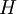$H$ is the enthalpy. The difference between the heat capacity at constant pressure and the heat capacity at constant volume is given by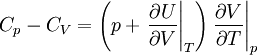$C_p -C_V = \left( p + \left. \frac{\partial U}{\partial V} \right\vert_T \right) \left. \frac{\partial V}{\partial T} \right\vert_p$

Sometimes the ratio of heat capacities is known as the adiabatic index: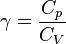$\gamma = \frac{C_p}{C_V}$

## Excess heat capacity

In a classical system the excess heat capacity for a monatomic fluid is given by subtracting the ideal internal energy (which is kinetic in nature)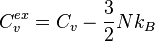$C_v^{ex} = C_v - \frac{3}{2}Nk_B$

in other words the excess heat capacity is associated with the component of the internal energy due to the intermolecular potential, and for that reason it is also known as the configurational heat capacity. Given that the excess internal energy for a pair potential is given by (Eq. 2.5.20 in ):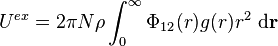$U^{ex} = 2\pi N \rho \int_0^{\infty} \Phi_{12}(r) g(r) r^2 ~{\rm d}{\mathbf r}$

where$\Phi_{12}(r)$ is the intermolecular pair potential and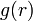$g(r)$ is the radial distribution function, one has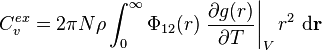$C_v^{ex} = 2\pi N \rho \int_0^{\infty} \Phi_{12}(r) \left. \frac{\partial g(r)}{\partial T} \right\vert_V r^2 ~{\rm d}{\mathbf r}$

For many-body distribution functions things become more complicated .

### Rosenfeld-Tarazona expression

Rosenfeld and Tarazona   used fundamental-measure theory to obtain a unified analytical description of classical bulk solids and fluids, one result being: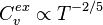$C_v^{ex} \propto T^{-2/5}$

## Liquids

The calculation of the heat capacity in liquids is more difficult than in gasses or solids . Recently an expression for the energy of a liquid has been developed (Eq. 5 of ):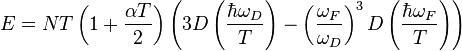$E = NT \left( 1 + \frac{\alpha T}{2}\right) \left( 3D \left( \frac{\hbar \omega_D}{T} \right) -\left( \frac{\omega_F}{\omega_D} \right)^3 D\left( \frac{\hbar \omega_F}{T}\right) \right)$

where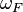$\omega_F$ is the Frenkel frequency,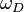$\omega_D$ is the Debye frequency,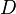$D$ is the Debye function, and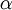$\alpha$ is the thermal expansion coefficient. The differential of this energy with respect to temperature provides the heat capacity.

## Solids

#### Debye

A low temperatures on has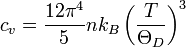$c_v = \frac{12 \pi^4}{5} n k_B \left( \frac{T}{\Theta_D} \right)^3$

where$k_B$ is the Boltzmann constant,$T$ is the temperature and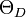$\Theta_D$ is an empirical parameter known as the Debye temperature.